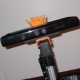# Extracting raw data from Kinect

Hello all,

I am new to ROS and I would like to know how to subscribe to a topic and save the data in python. I understand how to log data using rosbag or to echo the data on the topic, but I do not know how to save the data to a variable. I am trying to modify the tutorial code to simply display the raw data.

#!/usr/bin/env python
import rospy
from std_msgs.msg import String
def callback(data):

def listener():
rospy.init_node('listener', anonymous=True)
rospy.Subscriber("camera/rgb/image_color", String, callback)
#declares that your node subscribes to the chatter topic which is of type   s   std_msgs.msgs.String
rospy.spin()
#keeps your node from exiting until the node has been shutdown

if __name__ == '__main__':
listener()


Any help would be greatly appreciated.

-Sean

edit retag close merge delete

Sort by » oldest newest most votedYou might want to convert the sensor_msgs/Image datatype to an OpenCV image. The cv_bridge tutorial for Python shows how to do so: http://www.ros.org/wiki/cv_bridge/Tutorials/ConvertingBetweenROSImagesAndOpenCVImagesPython

The below code is an example from our SVN ( http://code.google.com/p/albany-ros-pkg ) that captures an image, blurs it, and displays it:

#!/usr/bin/env python
"""
Example code of how to convert ROS images to OpenCV's cv::Mat
This is the solution to HW2, using Python.

http://www.ros.org/wiki/cv_bridge
"""

import rospy

import cv
from cv_bridge import CvBridge, CvBridgeError

from std_msgs.msg import String
from sensor_msgs.msg import Image

class image_blur:

def __init__(self):
# initialize a node called hw2
rospy.init_node("hw2")

# create a window to display results in
cv.NamedWindow("image_view", 1)

# part 2.1 of hw2 -- subscribe to a topic called image
self.image_sub = rospy.Subscriber("image", Image, self.callback)

def callback(self,data):
""" This is a callback which recieves images and processes them. """
# convert image into openCV format
bridge = CvBridge()
try:
# bgr8 is the pixel encoding -- 8 bits per color, organized as blue/green/red
cv_image = bridge.imgmsg_to_cv(data, "bgr8")
except CvBridgeError, e:
# all print statements should use a rospy.log_ form, don't print!

# we could do anything we want with the image here
# for now, we'll blur using a median blur
cv.Smooth(cv_image, cv_image, smoothtype=cv.CV_MEDIAN, param1=31, param2=0, param3=0, param4=0)

# show the image
cv.ShowImage("image_view", cv_image)
cv.WaitKey(3)

if __name__ == '__main__':
image_blur()
try:
rospy.spin()
except KeyboardInterrupt:
print "Shutting down"
cv.DestroyAllWindows()

more